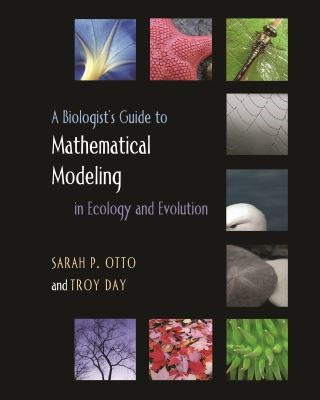## A Biologist's Guide to Mathematical Modeling in Ecology and Evolution• Description
• Author
• Info
• Reviews

### Description

Thirty years ago, biologists could get by with a rudimentary grasp of mathematics and modeling. Not so today. In seeking to answer fundamental questions about how biological systems function and change over time, the modern biologist is as likely to rely on sophisticated mathematical and computer-based models as traditional fieldwork. In this book, Sarah Otto and Troy Day provide biology students with the tools necessary to both interpret models and to build their own.

The book starts at an elementary level of mathematical modeling, assuming that the reader has had high school mathematics and first-year calculus. Otto and Day then gradually build in depth and complexity, from classic models in ecology and evolution to more intricate class-structured and probabilistic models. The authors provide primers with instructive exercises to introduce readers to the more advanced subjects of linear algebra and probability theory. Through examples, they describe how models have been used to understand such topics as the spread of HIV, chaos, the age structure of a country, speciation, and extinction.

Ecologists and evolutionary biologists today need enough mathematical training to be able to assess the power and limits of biological models and to develop theories and models themselves. This innovative book will be an indispensable guide to the world of mathematical models for the next generation of biologists.

• A how-to guide for developing new mathematical models in biology

• Provides step-by-step recipes for constructing and analyzing models

• Interesting biological applications

• Explores classical models in ecology and evolution

• Questions at the end of every chapter

• Primers cover important mathematical topics